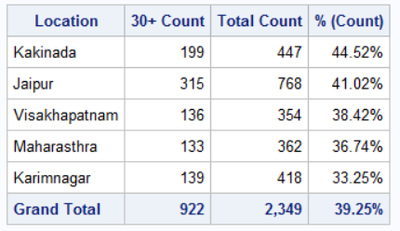## How can we sort data by PCT column from largest to smallest as it is computed column in proc report

``````TITLE height=3 color=BLUE J=LEFT "Branch Wise 1+ Count";
PROC REPORT DATA=WORK.FINAL_DPD LS=132 PS=60  SPLIT="/" CENTER ;
COLUMN  LOAN_BRANCH_BHEEM DPD TOTAL_COUNT PCT;
DEFINE  DPD / SUM FORMAT= 11. WIDTH=11    SPACING=2   CENTER "1+ Count" ;
DEFINE  LOAN_BRANCH_BHEEM / DISPLAY FORMAT= \$150. WIDTH=150   SPACING=2   LEFT "Branch";
DEFINE 	TOTAL_COUNT / SUM CENTER "Total Count";
DEFINE  PCT/computed format = percent8.2 CENTER "% (Count)";

RBREAK AFTER/SUMMARIZE;
COMPUTE AFTER;
LOAN_BRANCH_BHEEM="Grand Total";
if upcase(_BREAK_)="_RBREAK_" then do;
call define (_row_,'style','style=[background=#EDF2F9 foreground=#112277 fontweight=bold');
END;
ENDCOMP;

COMPUTE PCT;
PCT =_c2_/_c3_;
ENDCOMP;
RUN;``````
1 ACCEPTED SOLUTION

Accepted Solutions

## Re: How can we sort data by PCT column from largest to smallest as it is computed column in proc rep

Calculate the percentage in a preceding DATA step, so you can sort the result BY DESCENDING PCT.

Then run PROC REPORT without the COMPUTE.

6 REPLIES 6

## Re: How can we sort data by PCT column from largest to smallest as it is computed column in proc rep

Calculate the percentage in a preceding DATA step, so you can sort the result BY DESCENDING PCT.

Then run PROC REPORT without the COMPUTE.

## Re: How can we sort data by PCT column from largest to smallest as it is computed column in proc rep

 Grand Total 30053 196653

Many Thanks @Kurt_Bremser as I am not able to get grand total against two columns as I am using display as an option.

Request to opinion on this.

## Re: How can we sort data by PCT column from largest to smallest as it is computed column in proc rep

Pleas post usable example data which leads to this expected outcome, so I can verify any code suggestions.

## Re: How can we sort data by PCT column from largest to smallest as it is computed column in proc rep

 Location 30+ Count Total Count % (Count) Kakinada 199 447 44.52% Jaipur 315 768 41.02% Visakhapatnam 136 354 38.42% Maharasthra 133 362 36.74% Karimnagar 139 418 33.25% Grand Total 922 2349

@Kurt_Bremser  I am posting table which was asked earlier.

But everything comes right as it got sorted but Grand Total is not coming as I am expecting 30+ Count/Total Count.

## Re: How can we sort data by PCT column from largest to smallest as it is computed column in proc rep

Add the calculation to the COMPUTE block:

``````compute after;
LOAN_BRANCH_BHEEM = "Grand Total";
_c4_ = _c2_ / _c3_;
if upcase(_BREAK_)="_RBREAK_" then do;
call define (_row_,'style','style=[background=#EDF2F9 foreground=#112277 fontweight=bold');
end;
endcomp;``````

Just an idea, untested.

## Re: How can we sort data by PCT column from largest to smallest as it is computed column in proc rep

That is not data, looks like perhaps the report?
So first let's convert it into actual data so we have something to program with.

``````data have;
input Location :\$20. count total percent :percent.;
cards;
Jaipur  315 768 41.02%
Visakhapatnam 136 354 38.42%
Maharasthra 133 362 36.74%
Karimnagar  139 418 33.25%
;``````

Now we can write PROC REPORT code.  You can use the PERCENT column twice.  Once to GROUP the report so you can use it to order and again so the value is displayed.  You can use COMPUTE AFTER block to add the GRAND TOTAL row label and also to fix up the percent.

``````proc report data=have;
column percent location count total percent=x_percent ;
define percent / group descending order=internal noprint;
define location / group order=data ;
define count / sum '30+ Count' format=comma7.;
define Total / sum 'Total Count' format=comma7.;
define x_percent / '% (Count)' format=percent8.2 ;
rbreak after / summarize;
compute after;
location='Grand Total';
x_percent = (count.sum/total.sum);
call define (_row_,'style','style=[background=#EDF2F9 foreground=#112277 fontweight=bold');
endcomp;
run;``````

ResultIf you don't want the percent of 30+ day values on the Grand Total line then set it to missing instead.  You might want to set the system option MISSING to ' ' before hand so the value is not displayed as a period.

Discussion stats
• 6 replies
• 246 views
• 0 likes
• 3 in conversation